Trigonometry : Finding Sides

Example Questions

← Previous 1

Example Question #1 : Finding Sides

If the height of the stair is 2 ft, and the length of the stair is 3 ft, how long must the ramp be to cover the stair?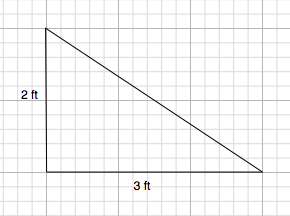2.5 ft

5 ft

3.6 ft

10 ft

13 ft

3.6 ft

Explanation:

Use the Pythagorean triangle to solve for the third side of the triangle.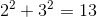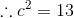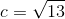Simplify and you have the answer: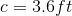Example Question #2 : Solving Triangles

A surveyor looks up to the top of a mountain at an angle of 35 degrees.  If the surveyor is 2400 feet from the base of the mountain, how tall is the mountain (to the nearest tenth of a foot)?

2135.8 feet

1376.6 feet

1966.0 feet

3427.6 feet

1680.5 feet

1680.5 feet

Explanation:

This is a typical angle of elevation problem.

From the question, we can infer that the ratio of the mountain height to the surveyor's horizontal distance from the mountain is equal to the tangent of 35 degrees - the mountain height is the "opposite side", while the horizontal distance is the "adjacent side".  In other words: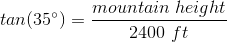Solving for the mountain height gives a vertical distance of 1680.5 feet.

Example Question #2 : Finding Sides

In a right triangle, the legs areand. What is the length of the hypotenuse?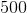Explanation:

Here, we can use the Pythagorean Theorem.

This states that the sum of the squares of the legs is equal to the square of the hypotenuse.

Thus, we plug into the formula to get our hypotenuse.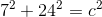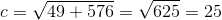Example Question #113 : Triangles

If the altitude of a triangle has a height of 5, and also bisects a 60 degree angle, what must be the perimeter of the full triangle?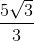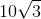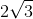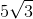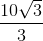Explanation:

The altitude of the triangle splits the 60 degree angle into two 30 degree angles.  Notice that this will create two right triangles with 30-60-90 angles.  This indicates that the full triangle is an equilateral triangle.

The altitude represents the adjacent side of the split triangles. The bisected angle is 30 degrees.  Find the hypotenuse of either split triangle.  This is the side length of the equilateral triangle.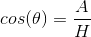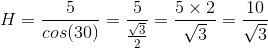Rationalize the denominator.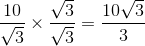Since the full triangle has three sides, multiply this value by three for the perimeter.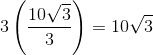Example Question #112 : Triangles

A horizontal ramp of unknown length meets the ground at angle of 20°. The height of the ramp is 6 feet and makes a right angle with the ground. What is the length of the ramp?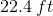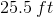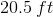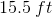Explanation:

The question is looking for the length of the ramp which would be the length of the part one would walk on and is also synonymous with the hypotenuse of our right triangle. With the given angle and opposite side we can use the sine function to solve for the length of the ramp because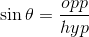.

We have all but the length of the hypotenuse.

Solve for the hypotenuse: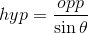Plug in the angles and sides given: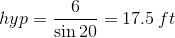Example Question #115 : Triangles

A zipline is attached from the edge of the roof of a 23 foot high building and meets the ground 23 feet from the base of the building. Find the angle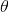of elevation the cable makes with the ground.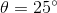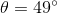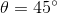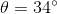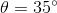Explanation:

Concerning the angle of elevation which we are to find, we know the side length opposite this angle and the side length adjacent to it.

We use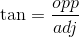.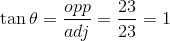Take the inverse of tan to find the corresponding angle: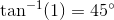A short cut for solving is to realize right triangles with equal sides must have two 45° angles!

Example Question #1 : Finding Sides

If the two legs of a right triangle are 5 and 7 respectively, what is the length of the hypotenuse?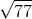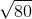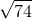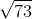Explanation:

Step 1: Recall the Pythagorean Theorem:, where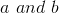are the legs of the triangle.

Step 2: Plug in the values that are given for the legs into the theorem: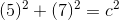Evaluate: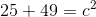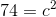Step 3: To find, take the square root of both sides: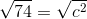Reduce (if possible):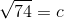The length of the hypotenuse is.

Example Question #121 : Triangles

In a right triangle, one of the legs has a length ofand a hypotenuse of. Find the length of the other side...Explanation:

Step 1: To find the missing side of a right triangle using the Pythagorean Theorem:.

Step 2: Substitute in the known values.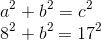Step 3: Solve for the missing variable by manipulating the equation to isolate the variable.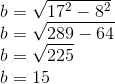Example Question #4 : Finding Sides

Find the length of.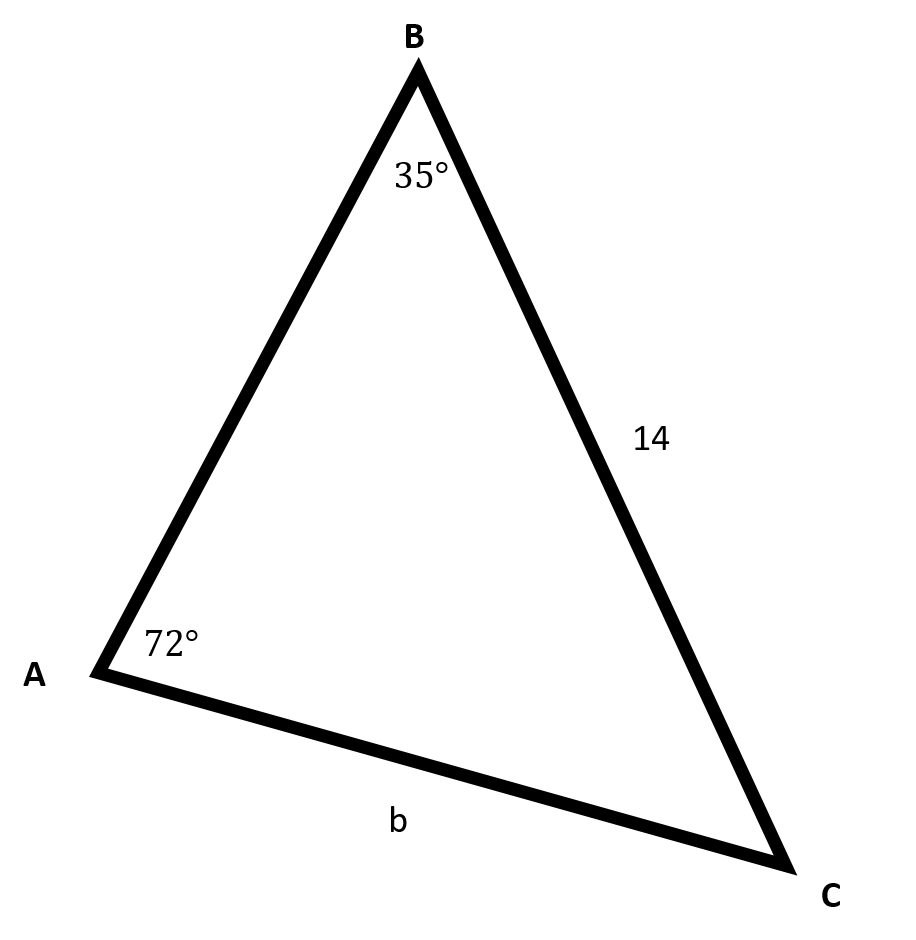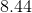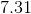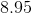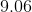Explanation:Recall the Law of Sines for a generic triangle, as shown above: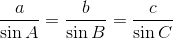Plug in the given values into the Law of Sines: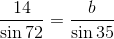Rearrange the equation to solve for: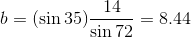Make sure to round to two places after the decimal.

Example Question #5 : Finding Sides

Find the length of side.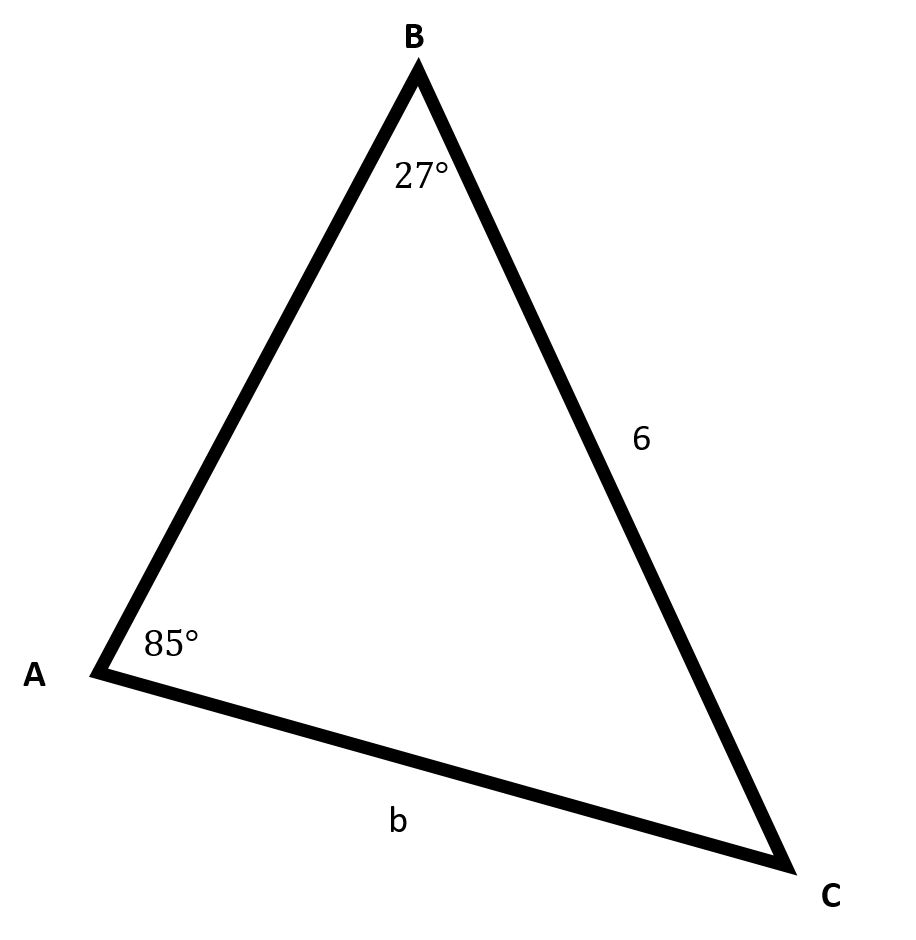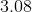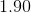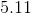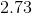Explanation:Recall the Law of Sines:Plug in the given values into the Law of Sines: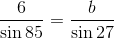Rearrange the equation to solve for: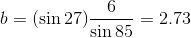Make sure to round to two places after the decimal.

← Previous 1